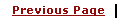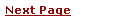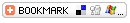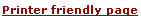C - Functions and PrototypesLearning C
C Function References
C Useful Resources# C - Using FunctionsA function is a module or block of program code which deals with a particular task. Making functions is a way of isolating one block of code from other independent blocks of code.

Functions serve two purposes.

• They allow a programmer to say: `this piece of code does a specific job which stands by itself and should not be mixed up with anyting else',

• Second they make a block of code reusable since a function can be reused in many different contexts without repeating parts of the program text.

A function can take a number of parameters, do required processing and then return a value. There may be a function which does not return any value.

You already have seen couple of built-in functions like printf(); Similar way you can define your own functions in C language.

Consider the following chunk of code

 ``` int total = 10; printf("Hello World"); total = total + l; ```

To turn it into a function you simply wrap the code in a pair of curly brackets to convert it into a single compound statement and write the name that you want to give it in front of the brackets:

 ```Demo() { int total = 10; printf("Hello World"); total = total + l; } ```

curved brackets after the function's name are required. You can pass one or more paramenters to a function as follows:

 ```Demo( int par1, int par2) { int total = 10; printf("Hello World"); total = total + l; } ```

By default function does not return anything. But you can make a function to return any value as follows:

 ```int Demo( int par1, int par2) { int total = 10; printf("Hello World"); total = total + l; return total; } ```

A return keyword is used to return a value and datatype of the returned value is specified before the name of function. In this case function returns total which is int type. If a function does not return a value then void keyword can be used as return value.

Once you have defined your function you can use it within a program:

 ```main() { Demo(); } ```

# Functions and Variables:

Each function behaves the same way as C language standard function main(). So a function will have its own local variables defined. In the above example total variable is local to the function Demo.

A global variable can be accessed in any function in similar way it is accessed in main() function.

# Declaration and Definition

When a function is defined at any place in the program then it is called function definition. At the time of definition of a function actual logic is implemented with-in the function.

A function declaration does not have any body and they just have their interfaces.

A function declaration is usually declared at the top of a C source file, or in a separate header file.

A function declaration is sometime called function prototype or function signature. For the above Demo() function which returns an integer, and takes two parameters a function declaration will be as follows:

 ```int Demo( int par1, int par2); ```

# Passing Parameters to a Function

There are two ways to pass parameters to a function:

• Pass by Value: mechanism is used when you don't want to change the value of passed paramters. When parameters are passed by value then functions in C create copies of the passed in variables and do required processing on these copied variables.

• Pass by Reference mechanism is used when you want a function to do the changes in passed parameters and reflect those changes back to the calling function. In this case only addresses of the variables are passed to a function so that function can work directly over the addresses.

Here are two programs to understand the difference: First example is for Pass by value:

 ``` #include /* function declaration goes here.*/ void swap( int p1, int p2 ); int main() { int a = 10; int b = 20; printf("Before: Value of a = %d and value of b = %d\n", a, b ); swap( a, b ); printf("After: Value of a = %d and value of b = %d\n", a, b ); } void swap( int p1, int p2 ) { int t; t = p2; p2 = p1; p1 = t; printf("Value of a (p1) = %d and value of b(p2) = %d\n", p1, p2 ); } ```

Here is the result produced by the above example. Here the values of a and b remain unchanged before calling swap function and after calling swap function.

 ```Before: Value of a = 10 and value of b = 20 Value of a (p1) = 20 and value of b(p2) = 10 After: Value of a = 10 and value of b = 20 ```

Following is the example which demonstrate the concept of pass by reference

 ```#include /* function declaration goes here.*/ void swap( int *p1, int *p2 ); int main() { int a = 10; int b = 20; printf("Before: Value of a = %d and value of b = %d\n", a, b ); swap( &a, &b ); printf("After: Value of a = %d and value of b = %d\n", a, b ); } void swap( int *p1, int *p2 ) { int t; t = *p2; *p2 = *p1; *p1 = t; printf("Value of a (p1) = %d and value of b(p2) = %d\n", *p1, *p2 ); } ```

Here is the result produced by the above example. Here the values of a and b are changes after calling swap function.

 ```Before: Value of a = 10 and value of b = 20 Value of a (p1) = 20 and value of b(p2) = 10 After: Value of a = 20 and value of b = 10 ```